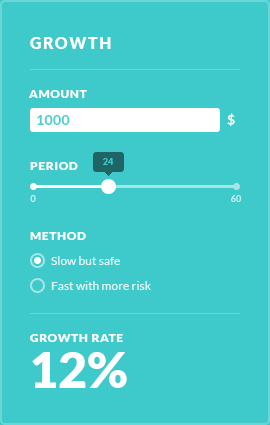# Investment calculator

The Simple Investment Calculator calculates how much money you will get each month of the year by calculating the interest rate of the loan that's been given out and adding it to the sum of the loan and dividing it by the number of months in the year.

### Formula

• #### #3 formula

Loan amount * (Interest / 100)

• #### Payment

#3 / 12 * (1.4/100)

### Works best for

It is a good calculator for a basic estimate of how much your invesment may pay off with the given interest rate or how much you will have to pay for the loan you want to take out.